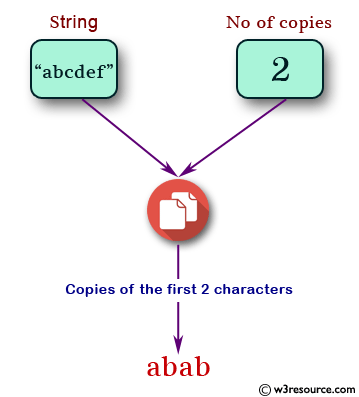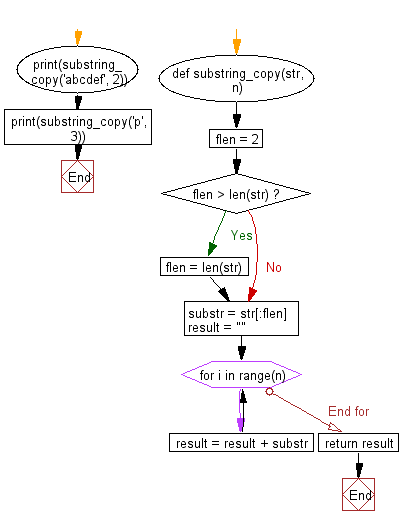﻿ Python: n (non-negative integer) copies of the first 2 characters of a given string - w3resource# Python: n (non-negative integer) copies of the first 2 characters of a given string

## Python Basic: Exercise-23 with Solution

Write a Python program to get the n (non-negative integer) copies of the first 2 characters of a given string. Return the n copies of the whole string if the length is less than 2.

Pictorial Presentation:Sample Solution:-

Python Code:

``````def substring_copy(str, n):
flen = 2
if flen > len(str):
flen = len(str)
substr = str[:flen]

result = ""
for i in range(n):
result = result + substr
return result
print(substring_copy('abcdef', 2))
print(substring_copy('p', 3));
```
```

Sample Output:

```abab
ppp
```

Flowchart:## Visualize Python code execution:

The following tool visualize what the computer is doing step-by-step as it executes the said program:

Python Code Editor:

Have another way to solve this solution? Contribute your code (and comments) through Disqus.

What is the difficulty level of this exercise?

Test your Python skills with w3resource's quiz

﻿

## Python: Tips of the Day

Try-catch-else construct:

```try:
foo()
except Exception:
print("Exception occured")
else:
print("Exception didnt occur")
finally:
print("Always gets here")
```